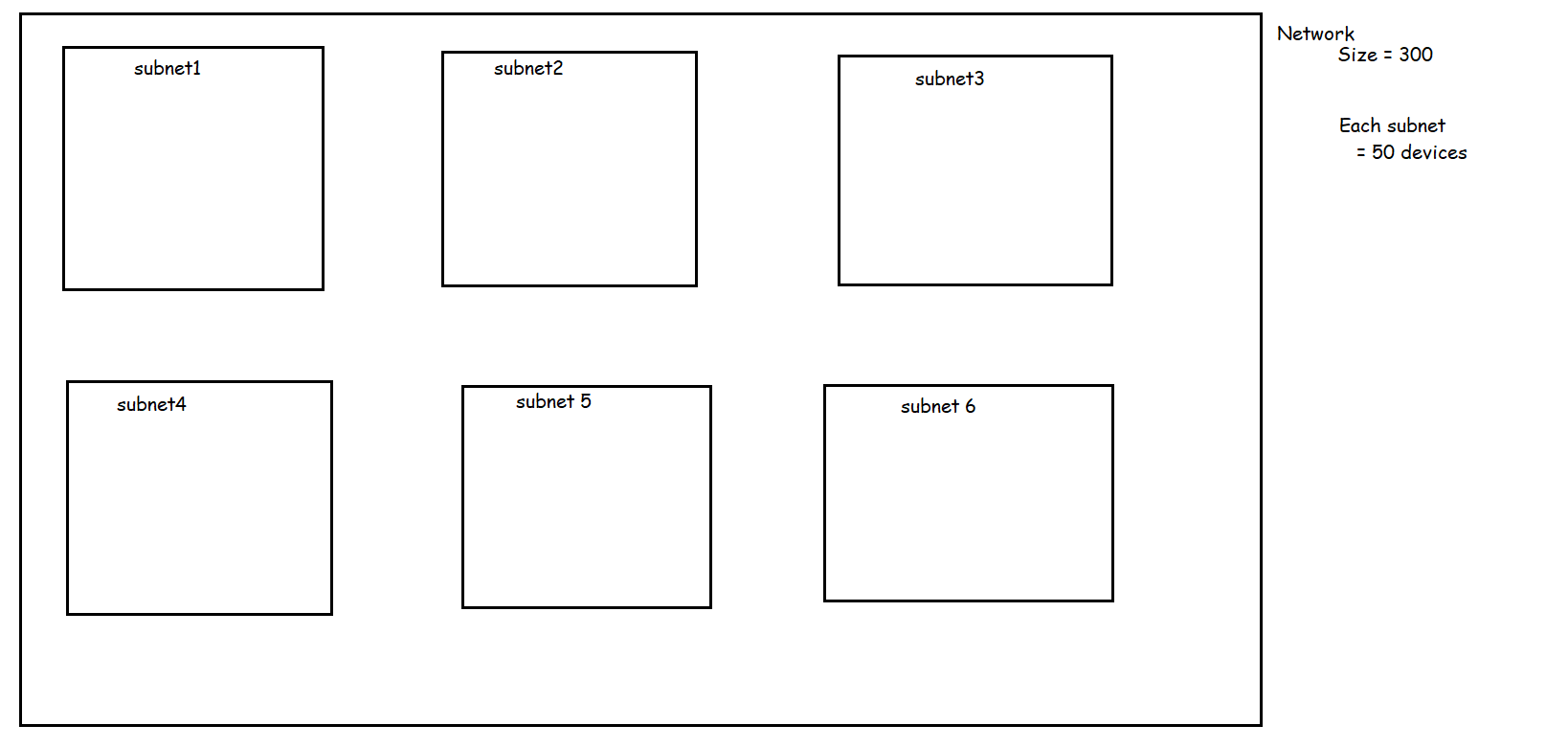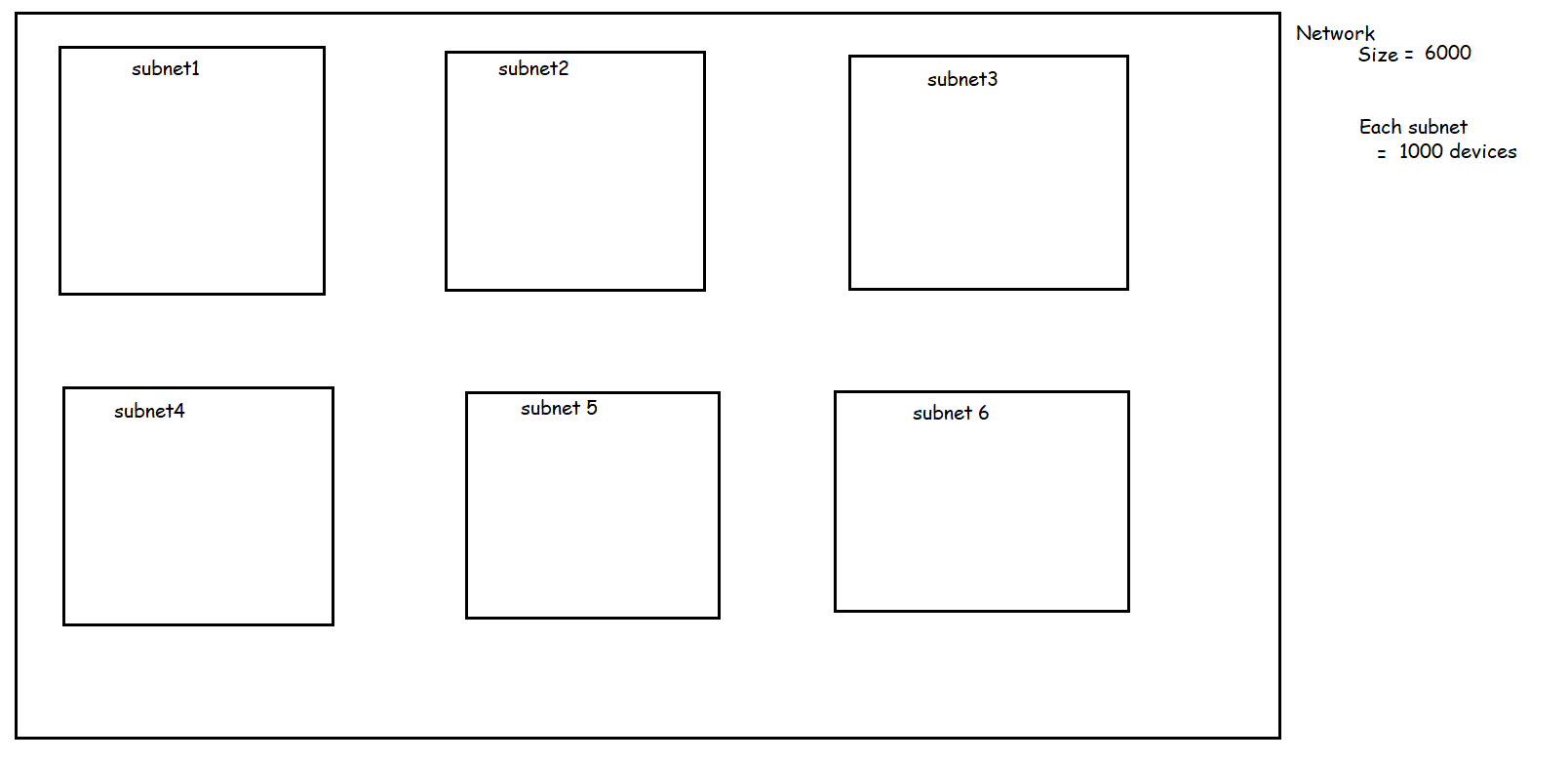### Networking contd

• Lets create a private network of size 10000 devices
``````2^n ~= 10000
number of zeros = 14
number of ones = 32-14 = 18
subnet mask : 11111111.11111111.11000000.00000000
subnet mask: 255.255.192.0
``````
• My private network can be from any of the cidr ranges
• 10.0.0.0/8
• 172.16.0.0/12
• 192.168.0.0/16
• So the private network will be
``````192.168.0.0/18
or
10.0.0.0/18
or
172.16.0.0/18
``````
• Lets create a private network of size 100000 devices
``````2^n ~= 100000
number of zeros => 17
number of ones => 32-17 = 15
to choose a range
10.0.0.0/15
or
172.16.0.0/15
``````
• Now since we know how to create a network, we should also look into how to break the network into smaller networks called as subnets

#### Scenario-1: So lets start with the following scenario

• network• network range
``````2^n ~= 1000
number of zeros = 10
number of ones = 32-10 =22
since this is private network
ip: 192.168.0.0/22
sm: 11111111.11111111.11111100.00000000
sm: 255.255.252.0
``````
• Now we need to create subnets in this network. Each subnet size is 250 devices
``````2^n ~= 250
number of zeros = 8
number of ones = 32-8 = 24
sm: 11111111.11111111.11111111.00000000
sm: 255.255.255.0

subnet sm : 11111111.11111111.11111111.00000000
network sm: 11111111.11111111.11111100.00000000

for subnets we have two bits
xx
00
01
10
11

subnet1
00 => 192.168.0.0/24

subnet 2 =>
01 => 192.168.1.0/24

subnet 3 =>
10 => 192.168.2.0/24

subnet 4 =>
11 => 192.168.3.0/24
``````

#### Scenario 2

• Network requirements• network size is 4000
``````2^n ~= 4000
number of zeros (n) = 12
number of ones => 32-12 => 20

sm: 11111111.11111111.11110000.00000000
sm: 255.255.240.0
``````
• Each subnet should have 1000 devices
``````2^n ~=1000
number of zeros (n) = 10
number of ones => 32-10 = 22
sm: 11111111.11111111.11111100.00000000
``````
• Lets compare subnet mask of subnet and network
``````subnet:  11111111.11111111.11111100.00000000
network: 11111111.11111111.11110000.00000000
so positons
xx00
``````
• Lets choose a private range for network
``````10.10.0.0/20
``````
• Now lets try to come up with cidr ranges for the subnets
``````xx00
first subnet => 0000
subnet 1: 10.10.0.0/22

subnet2 => 0100 => 10.10.4.0/22

subnet3 => 1000 => 10.10.8.0/22

subnet4 => 1100 => 10.10.12.0/22
``````

### Scenario 3:

• Networking requirements• network size is 300 devices
``````2^n ~= 300
number of zeros (n) = 9
number of 1's will be 32-9 => 23
``````
• network cidr range and subnet mask
``````ip: 192.168.0.0/23
sm: 11111111.11111111.11111110.00000000
sm: 255.255.254.0

``````
• each subnet should be of size 50
``````2^n ~= 50
n = 6 =>
number of ones => 32-6 => 26

``````
• subnet mask of each subnet will be
``````sm: 11111111.11111111.11111111.11000000
``````
• now lets compare subnet mask of network and subnet
``````subnet : 11111111.11111111.11111111.11000000
network: 11111111.11111111.11111110.00000000
x.xx000000
``````
• if we have 3 positions
``````xxx
000
001
010
011
100
101
110
111
``````
• lets find cidr ranges for subnets
``````x.xx000000
subnet 1 => 000=> 0.00000000 => 0.0 => 192.168.0.0/26
x.xx000000
subnet2=> => 001 =>0.01000000 => 0.64 => 192.168.0.64/26
x.xx000000
subnet 3 => 010 => 0.10000000 => 0.128 => 192.168.0.128/26
x.xx000000
subnet 4 => 011 => 0.11000000 => 0.192 => 192.168.0.192/26
x.xx000000
subnet 5 => 100 => 1.00000000 => 1.0 => 192.168.1.0/26
x.xx000000
subnet 6 => 101 => 1.01000000 => 1.64 => 192.168.1.64/26
``````

#### Scenario 4: Exercise

• Network requirementsThis site uses Akismet to reduce spam. Learn how your comment data is processed.